# Complete Vism  (Read 4520 times)

Started by Messatsu, July 04, 2004, 12:34:11 am

#### Messatsu##### Complete Vism
New #1  July 04, 2004, 12:34:11 am
••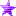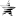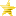• In after lock
Recording helper setup

Code:
`;___________________________________________________________________[Statedef 500];                // custom combo functionanim = 11111[State Pos X]type     = helpertrigger1 = !timeid       = 501stateno  = 501[State Pos Y]type     = helpertrigger1 = !timeid       = 502stateno  = 502[State Anim]type     = helpertrigger1 = !timeid       = 503stateno  = 503[State Stateno]type     = helpertrigger1 = !timeid       = 504stateno  = 504[State Facing]type     = nulltrigger1 = !(root, hitpausetime)trigger1 = fvar((floor(var(0) / 2))) := root, facing[State Clone]type     = varsettrigger1 = !timevar(1)   = 1[State Next]type     = varsettrigger1 = !timevar(2)   = 2[State Vism]type     = helpertrigger1 = numhelper(666) < 1trigger1 = time > root, var(15) - 2trigger1 = helper(504), var((var(2))) != [0, 110)trigger1 = helper(504), var((var(1))) != helper(504), var((var(2)))postype  = leftpos      = helper(500), pos x - helper(501), var((var(2))) + 160, helper(500), pos y - helper(502), var((var(2)))id       = 666facing   = floor(fvar((floor(var(2) / 2))))stateno  = helper(504), var((var(2)))ignorehitpause = 1[State Modify]type     = varaddtrigger1 = !(root, hitpausetime)var(0)   = 1[State Modify]type     = varaddtrigger1 = !(root, hitpausetime)var(1)   = 1[State Modify]type     = varaddtrigger1 = !(root, hitpausetime)var(2)   = 1[State Train]type     = varsettrigger1 = var(0) = root, var(15)var(0)   = 0[State Train]type     = varsettrigger1 = var(1) = root, var(15)var(1)   = 0[State Train]type     = varsettrigger1 = var(2) = root, var(15)var(2)   = 0[State X_X]type     = destroyselftrigger1 = power = 0 || root, movetype = Hignorehitpause = 1;___________________________________________________________________[Statedef 501]anim = 11111[State 501]type     = nulltrigger1 = !(root, hitpausetime)trigger1 = var(( helper(500), var(0) )):= floor(helper(500), pos x - root, pos x)[State 501]type     = nulltrigger1 = !(root, hitpausetime)trigger1 = helper(500), var(0) = [0,30)trigger1 = fvar(( helper(500), var(0) )):= root, vel x[State X_X]type     = destroyselftrigger1 = numhelper(500) = 0ignorehitpause = 1;___________________________________________________________________[Statedef 502]anim = 11111[State 502]type     = nulltrigger1 = !(root, hitpausetime)trigger1 = var(( helper(500), var(0) )):= floor(helper(500), pos y - root, pos y)[State 502]type     = nulltrigger1 = !(root, hitpausetime)trigger1 = helper(500), var(0) = (29,60)trigger1 = fvar(( helper(500), var(0) - 30 )):= root, vel x[State X_X]type     = destroyselftrigger1 = numhelper(500) = 0ignorehitpause = 1;___________________________________________________________________[Statedef 503]anim = 11111[State 503]type     = nulltrigger1 = !(root, hitpausetime)trigger1 = var(( helper(500), var(0) )):= root, anim[State 503]type     = nulltrigger1 = !(root, hitpausetime)trigger1 = helper(500), var(0) = [0,30)trigger1 = fvar(( helper(500), var(0) )):= root, vel y[State X_X]type     = destroyselftrigger1 = numhelper(500) = 0ignorehitpause = 1;___________________________________________________________________[Statedef 504]anim = 11111[State 504]type     = nulltrigger1 = !(root, hitpausetime)trigger1 = var(( helper(500), var(0) )):= root, stateno[State 504]type     = nulltrigger1 = !(root, hitpausetime)trigger1 = helper(500), var(0) = (29,60)trigger1 = fvar(( helper(500), var(0) - 30 )):= root, vel y[State X_X]type     = destroyselftrigger1 = numhelper(500) = 0ignorehitpause = 1;___________________________________________________________________`

Note: untested on teh H4X0430 WinMugen.Many people risk their lives everyday by having Mugen.
Last Edit: July 04, 2004, 12:38:54 am by SirROXaLot

#### Messatsu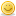##### Re:Complete Vism
New #2  July 04, 2004, 12:36:09 am
••• In after lock

STATES LISTED AS -4 NEED TO BE IN EVERY STATE THAT THE CLONE CAN POSSIBLY ENTER.

Typically, custom combos have different delays.  Your initialization should reflect this by modifying root, var(15):

Code:
`[State Vism]type       = helpertriggerall = numhelper(500) = 0trigger1   = command = "a"trigger1   = var(15):= 12trigger2   = command = "b"trigger2   = var(15):= 36trigger3   = command = "c"trigger3   = var(15):= 60stateno    = 500id         = 500postype    = p1pos        = 0,0`

;_________________________________________________ __________________
Disable all afterimage controllers by using a numhelper(500) = 0 triggerall except for one like this in the player's initialization state:

Code:
`[State Chaser]type     = afterimagetrigger1 = numhelper(500) = 1 && !ishelpertime     = 99999999length   = var(15)timegap  = 1framegap = floor(var(15) / 3)palcontrast = 144, 0, 256`

;_________________________________________________ __________________
Palfx cannot apply to helpers so you can leave the clone the same color as the player but adjust the sprpriority in the statedef shortcut somewhat like this:

Code:
`[Statedef 666]...sprpriority = ifelse(ishelper, -1, 2)`

Or to apply a fake palfx that has the clone visually delayed by 1 tick:

Code:
`[State -4]type     = assertspecialtrigger1 = ishelperflag     = invisible[State -4]type     = afterimagetrigger1 = ishelpertime     = 999999length   = 2timegap  = 1framegap = 1palcontrast = 96, 96, 256`

;_________________________________________________ __________________
The clone cannot be hit by anything:

Code:
`[State -4]type     = nothitbytrigger1 = ishelpertime     = 2value    = AA, AP, AT`

;_________________________________________________ __________________
This allows for the clone to combo:

Code:
`[State -4]type     = changestatetrigger1 = ishelpertrigger1 = helper(666), stateno != helper(504), var(( helper(500), var(2) ))trigger1 = helper(504), var(( helper(500), var(2) )) != [0,110)value    = helper(504), var(( helper(500), var(2) ))`

There will result in a slight error from the helper missing an attack where the player made contact resulting in a completely different position for the clone.  To fix this and use a changestate, you need to apply constant position adjustment:

Code:
`[State -4]type     = possettrigger1 = ishelperpostype  = p1x        = helper(500), pos x - helper(501), var((var(1))) + 160y        = helper(500), pos y - helper(502), var((var(1)))`

Alternatively, you can use a destroyself using similar restrictions to the changestate and allow the respawning in the correct position to be handled by the helper, 500.  I have found this fairly unreliable so far, but would be more efficient.

;_________________________________________________ __________________
Aerial states need to have their velocity adjusted.  Otherwise they start with nothing and sink to the ground.

Code:
`[State -4]type     = velsettrigger1 = ishelper && !timetrigger1 = helper(500), var(2) = [0,30)X        = helper(501), fvar( helper(500), var(2) )Y        = helper(503), fvar( helper(500), var(2) )[State -4]type     = velsettrigger1 = ishelper && time = 0trigger1 = helper(500), var(2) = (29,60)X        = helper(502), fvar( helper(500), var(2) - 30 )Y        = helper(504), fvar( helper(500), var(2) - 30 )`

;_________________________________________________ __________________
When two states are followed by each other, the second state won't be entered by the clone.  To avoid this, make the states wheel, if in state 200, then go to the exact same state 201 (only difference in 200 and 201 is stateno) given conditions to go into a state.

;_________________________________________________ __________________
States 0 and 11 need destroyselfs set.Many people risk their lives everyday by having Mugen.
Last Edit: July 04, 2004, 01:15:51 am by SirROXaLot

#### Messatsu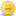##### Re:Complete Vism
#3  July 04, 2004, 12:37:53 am
••• In after lock
Necessary properties of Vism that I won't cover in this tutorial:

-The player cannot block during this time period
-Hit velocity doubles for fairness.
-Normals chain MVC style.
-Specials can limitlessly cancel into specials except into the same attack.
-Charging attacks no longer need to be charged.
-Projectile of the helper doesn't affect the spawning of the player's projectiles. For example, Shin Gouki in SFZ3U can throw four aerial hadokens on the screen.
-More than half of a full power bar is necessary to initialize.
-Power is drained every tick.
-You cannot perform a super attack.Many people risk their lives everyday by having Mugen.

#### Messatsu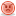##### Re:Complete Vism
#4  July 04, 2004, 12:38:24 am
••• In after lock
Known issues:
-Y position is off at times. Mostly following a jumping attack.
-The clone has a one tick lag if you want them colored correctly.
-Sprpriority of the clone is shifty during hits or other situations.Many people risk their lives everyday by having Mugen.